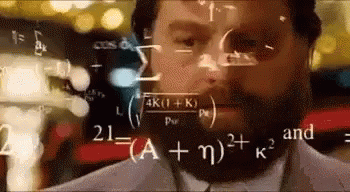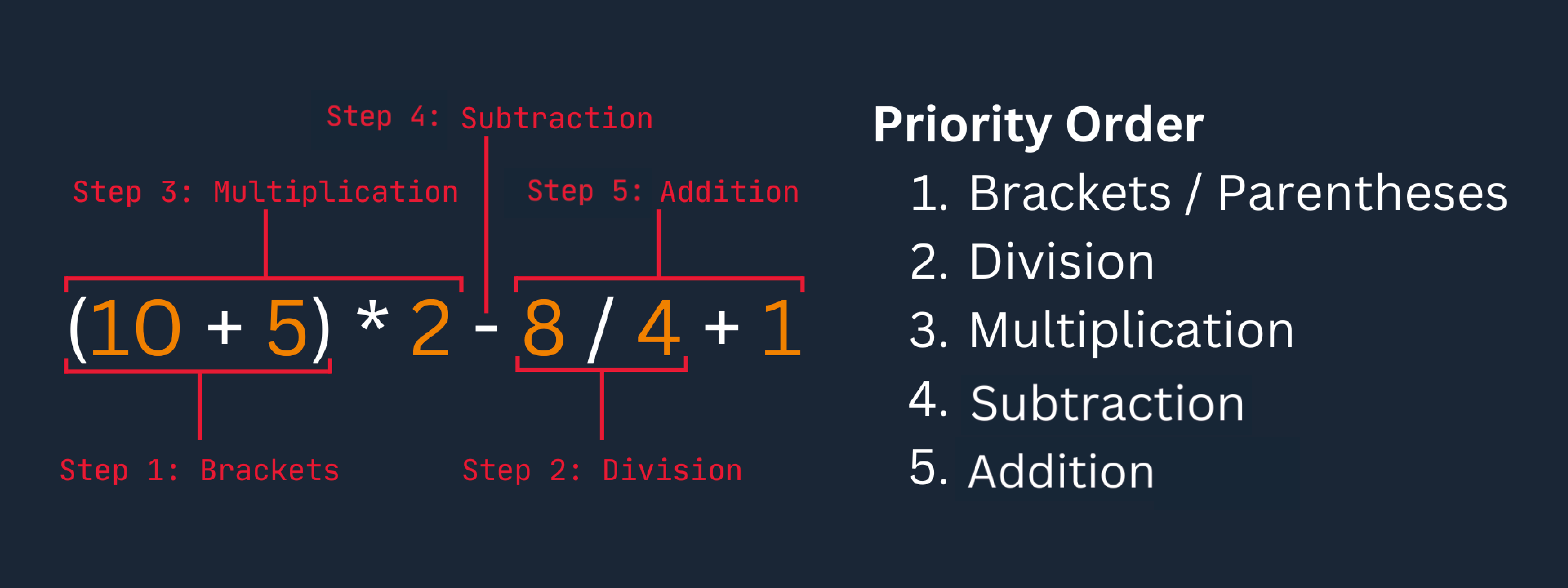Course Content

Java Basics

##Mathematical Operations in Java### Operators

In the previous chapter, we observed that we used basic mathematical operators such as `+`, `-`, `/`, and `*`. These operators are familiar to us from calculators or mathematics; you can also use them in a code editor.

Let's go through the basics:

• `+` – addition;
• `-` – subtraction;
• `/` – division;
• `*` – multiplication.You can use these four fundamental operators with numerical data types (`byte`, `short`, `long`, `float`, `double`).

Note

If you have gone through the previous chapter, you understand you can use these operators with `char`, but we won't focus on that now.

Let's explore the usage of these operators with an example code:Main.javaAs we can see, the variable `res` holds the value 27, which is the sum of 10 and 17.

Let's consider a few more examples:Main.javaWe can use both numbers and variables in our operations. However, it's worth remembering that creating multiple variables adds to the `stack memory`. Therefore, it's recommended to use regular numbers when possible. Additionally, we can observe that operator precedence is followed. Operations inside parentheses are performed first, followed by multiplication or division, and then addition or subtraction.

In this way, we can perform simple operations with different numeric data.

Note

It's also worth mentioning integer division with remainder. By default, Java will round the result to the nearest integer value. We can address this issue by changing the variable to a floating-point data type.

# Order of Operations

Java follows the basic principles of mathematics, and operations also have an order of execution. Let's take a look at an example:main.javaHere we arrived at the result by performing operations sequentially. Let's take a look at the order:So, you can prioritize the execution of operations using regular parentheses, just like in arithmetic.

Now, let's move on to a simple exercise to reinforce the material.

Try writing code that calculates the sum of the first and second numbers and then divides the result by the third number:Main.java• 1. Floating-point data types in Java are `double` and `float`; you can use either;
• 2. Also, you can use type casting here.
``````
package com.example;

public class Main {
public static void main(String[] args) {
int firstNumber = 9;
int secondNumber = 4;
double thirdNumber = 2;
double result = (firstNumber + secondNumber) / thirdNumber;
System.out.println(result);
}
}
``````Everything was clear?

Section 2. Chapter 4